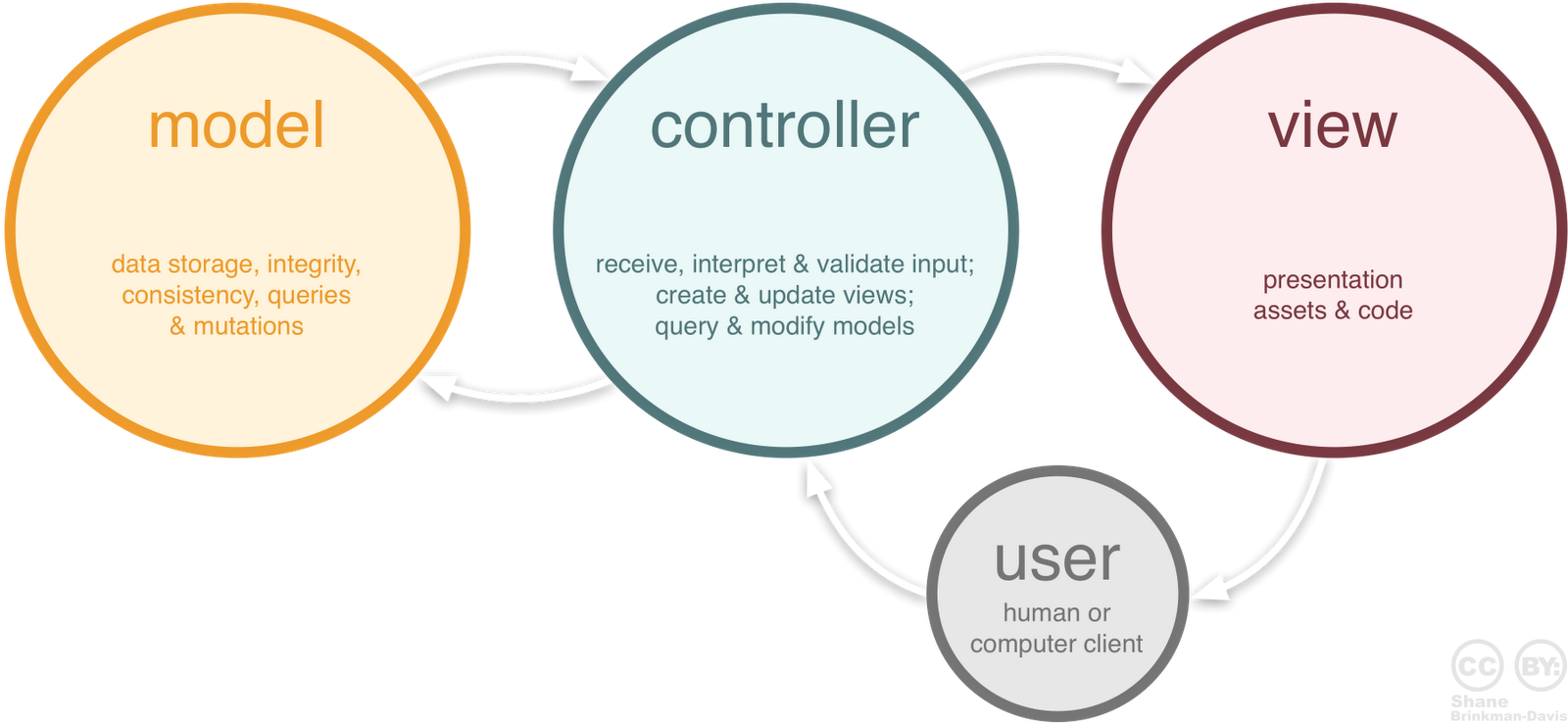# Effective Modern C++:constexpr, thread safe

constexpr is more strict than const

int a;
const b = a;//correct
constexpr c = a;//wrong, because a has no value yet


When declaring a constexpr, it should be assigned with a already existed value.

you can get compile-time result when using constexpr function

# Effective Modern C++:alias,enum,override

### Alias is preferred to typedef

It is clearer:

typedef void (*FP)(int, const std::string&); // old way: typedef
using FP = void (*)(int, const std::string&); // new way: alias


It support template:

template<typename T>
using MyAllocList = std::list<T, MyAlloc<T>>;


### enum is preferred to be scoped

Comparison between the old and new way:

# Data binding of ListBox and string list in C#

### DataBinding and MVVM Pattern

When dealing with GUI, usually we will use the MVC pattern, which means we manage a set of data as Model, the model will be demonstrated as View and the Contoller controls the logic of showing the data:The main problem of this pattern is that most of the controller’s works are simple “get” and “set” functions between the data and the gui Like:

# Effective Modern C++:decltyle,use {}

### decltype用法:

In C++11, perhaps the primary use for decltypeis declaring function templates where the function’s return typedepends on its parameter types.

decltype 用于获得某个未知变量的类型，在什么情况下我们不知道变量的类型呢？ 当然是使用template或auto的时候：

template<typename T>
void funcValue(T param)
{
auto subParam = param;
decltype(auto) subSubParam = subParam;
}


# Effective Modern C++:Template and auto

The type deduction of Template and auto

The type deduction of template will ignore one level of reference and pointer

Example 1:

Input argument as reference

template<typename T>
void f(T& param);

int x = 27; // x is an int
const int cx = x; // cx is a const int
const int& rx = x; // rx is a reference to x as a const int
f(x);// T is int
f(cx);// T is const int, param's typeis const int&
f(rx);// T is const int, param's type is const int&


# PCA and Face Recognition - Eigen Face

PCA (Principal component analysis), just as its name shows, it computes the data set’s internal structure, its “principal components”.

Considering a set of 2 dimensional data, for one data point, it has 2 dimensions $$x_1$$ and $$x_2$$ . Now we get n such data points . What is the relationship between the first dimension $$x_1$$ and the second dimension $$x_2$$ ? We compute the so called covariance:

$cov(x_1,x_2)=\frac\sum_{i=1}^n{(x_1^i-\overline{x_1})(x_2^i-\overline{x_2})} }{n-1$

the covariance shows how strong is the relationship between $$x_1$$ and $$x_2$$. Its logic is the same as Chebyshev’s sum inequality:

# Switch to another window using C#

[DllImport("user32.dll")]
public static extern void SwitchToThisWindow(IntPtr hWnd,bool turnon);
String ProcWindow = "wechat";
private void switchToWechart()
{
Process[] procs = Process.GetProcessesByName(ProcWindow);
foreach (Process proc in procs)
{
//switch to process by name
SwitchToThisWindow(proc.MainWindowHandle, true);
}
}


# HoG Feature

HoG (Histograms of Oriented Gradients) feature is a kind of feature used for human figure detection. At an age without deep learning, it is the best feature to do this work.

Just as its name described, HoG feature compute the gradients of all pixels of an image patch/block. It computes both the gradient’s magnitude and orientation, that’s why it’s called “oriented”, then it computes the histogram of the oriented gradients by separating them to 9 ranges.

One image block (upper left corner of the image) is combined of 4 cells, one cell owns a 9 bins histogram, so for one image block we get 4 histograms, and all these 4 histograms will be flattened to one feature vector with a length of 4x9. Compute the feature vectors for all blocks in the image, we get a feature vector map.

Taking one pixel (marked red) from the yellow cell as an example: compute the $$\bigtriangledown_x$$ and $$\bigtriangledown_y$$ of this pixel, then we get its magnitude and orientation(represented by angle). When calculating the histogram, we vote its magnitude to its neighboring 2 bins using bilinear interpolation of angles.

Finally, when we get the 4 histograms of the 4 cells, we normalize them according to the summation of all the 4x9 values.

The details are described in the following chart: